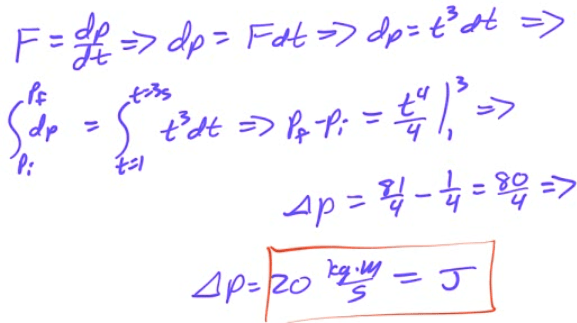# Momentum

• Momentum is a vector describing how difficult it is to stop a moving object

• Total momentum is the sum of individual momenta

•• Units are kg·m/s or N·s

# Example 1: Changing Momentum

• An Aichi D3A bomber mass (3600 kg) departs from its aircraft carrier with a velocity of 85 m/s due east. What is its momentum?• After it drops its payload, its new mass is 3000 kg and it attains a cruising speed of 120 m/s . What is its new momentum?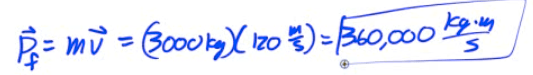# Impulse

• As you observed in the previous problem, momentum can change

• A change in momentum is known as an impulse (J)

•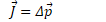# Example 2: Impulse

• The D3A bomber, which had a momentum of 3.6e5 kg·m/s, comes to halt on the ground. What impulse is applied?# Relationship Between Force and Impulse

•# Example 3: Force from Momentum

• The momentum of an object as a function of time is given by p=kt2, where k is a constant. What is the equation for the force causing this motion?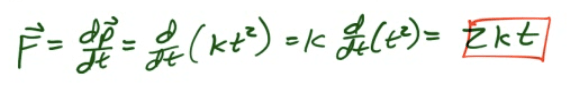# Impulse-Momentum Theorem# Example 4: Impulse-Momentum

• A 6-kg block, sliding to the east across a horizontal, frictionless surface with a momentum of 30 kg·m/s, strikes an obstacle. The obstacle exerts an impulse of 10 N·s to the west on the block. Find the speed of the block after the collision.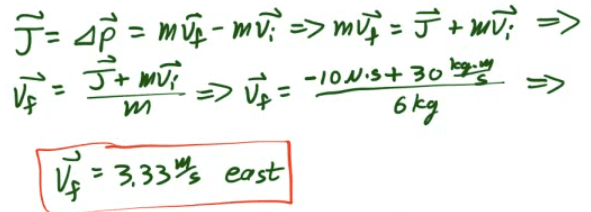# Example 5: Water Gun

• A girl with a water gun shoots a stream of water than ejects 0.2 kg of water per second horizontally at a speed of 10 m/s. What horizontal force must the girl apply on the gun in order to hold it in position?# Impulse from F-t Graphs

• Impulse is the area under a Force-time graph

• Impulse is equivalent to a change in momentum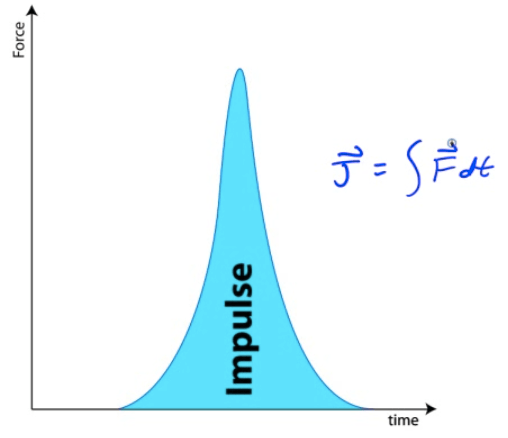# Example 6: Impulse from Force

• A force F(t)=t3</sup is applied to a 10 kg mass. What is the total impulse applied to the object between 1 and 3 seconds?